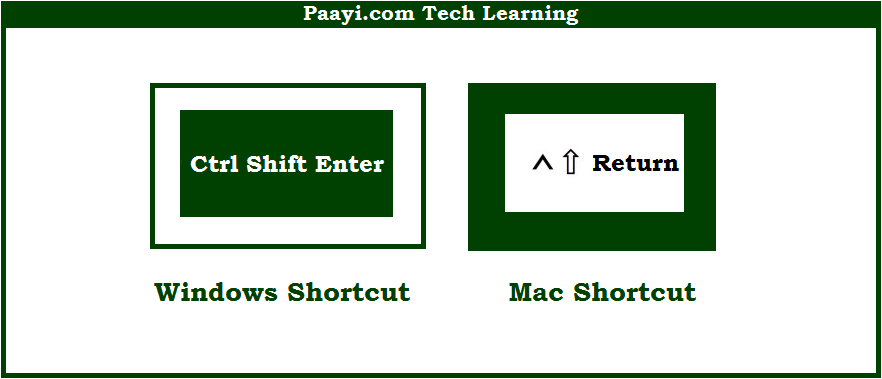# Learn How to Enter Array Formula With Shortcut in Microsoft Excel

Written by | 0 Comments | 491 Views

In this article, you will learn how to Enter Array Formula with a shortcut in Microsoft Excel. You will also get to know the shortcut to Enter Array Formula on Mac. The easy key combinations will make you operate Microsoft Excel smoothly.

Enter Array Formula With Shortcut in Microsoft Excel

Here we will show you how to enter the array formula in Microsoft Excel. Like many other programs Microsoft Excel also uses similar key combinations while using shortcuts.

You can use the key combinations given below to enter the array formula using the shortcut. With this shortcut, you can able to enter an array formula.  You will notice that the formula will be surrounded by the curly brackets when you enter an array formula like - {formula}. So, here we go:

## How to Enter Array Formula With Shortcut in Microsoft Excel?It is used to enter the array formula in Microsoft Excel. With this shortcut, you can able to enter an array formula.  You will notice that the formula will be surrounded by the curly brackets when you enter an array formula like - {formula}. This way you can easily enter the array formula using this shortcut.